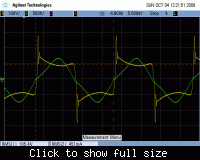# Finding peak lamp current in electronic CFL ballast

Status
Not open for further replies.

#### eem2am

##### BannedHello,

I am trying to find out how to find the maximum current that will occur for the LRES inductor in this CFL schematic.

Please could I be assisted to do this.?

CFL Schematic
*************I must know this so I can ensure that this inductor does not saturate.

Mains = 265VAC
LRES = 2.3mH
CRES = 6.8nF
Flourescent Tube ON’ resistance, R = 228 Ohms.

Now:-
iL(t) = inductor current at time t
iR(t) = Resistor current at time t
iC(t) = Capcitor current at time t

vL(t) = inductor voltage at time t
vR(t) = Resistor voltage at time t
vC(t) = Capcitor voltage at time t

V = Step input voltage of 188V at t=0
Z = impedance of L in series with parallel RC.

To cut a long story short………

…..this problem boils down to an inductor (LRES) in series with a parallel RC (where R = 227.8R and C= 6.8nF)………

That is, this circuit here
********************
LRC circuitA voltage of 188V appears across this LRC network at t = 0 (C initially uncharged and I(L) = 0 at t = 0.

…so here is the situation on LTSpice, with the step input of 188V, and the rising inductor current…….

*************It is then needed to solve (by calculation, not simulation) for what current appears in the inductor (LRES) at t=16us.

(This is due to 16us being the bridge half period time at the ‘RUN’ frequency)

Here is the circuit to be solved as above…..

L in series with parallel RC Circuit
**********I believe the quickest way to do this is to use Laplace Transforms.

(Using Kirchoffs Laws…….iL(t) = iR(t) + iC(t)

…….iL(t) = vC(t) / R + C * dvC(t) / dt

differentiating both sides with respect to t and rearranging……

[V - vc(t)] / L = C * [d^2]vc(t)/[dt]^2 + d.vc(t)/dt (1)

rearranging…

V/L = C * [d^2]vc(t)/[dt]^2 + d.vc(t)/dt + vc(t)/L (2)

This is then converted to the S domain…………

V/sL = C( [s^2]Vc(S) ) + s Vc(S) + Vc(S) / L (3)

Rearranging………

Vc(S) = V / { s * (CL[s^2] + sL + 1) } (4)

I have somehow got to convert the above equation (4) to a standard Laplace Transform so that I can convert back to one of the time-domain solutions as given in standard Laplace tables.

Unfortunately I am struggling with this.

I believe I must use Partial Fractions to help, but the ……………

“s * (CL[s^2] + sL + 1) “

…..term in the denominator of (4) appears to defy all the partial fraction forms given in my maths book.

Any help to get equation (4) into a standard Laplace Transform much appreciated.

When I have solved equation (4) I will have an expression for vC(t). (Cap voltage)

-I will then be able to do Q = C * vC(t) to find the Q (i.e. charge) in the cap at time t .

the current in the capacitor will be iC(t) = dQ/dt

current in the resistor will be iR(t) = vC(t)/R

then the required inductor current , iL(t) will be iR(t) + iC(t)
Anyway, I have not been able to convert equation (4) to the time domain so ,

%%%%%%%%%%%%%%%%%%%%%%%%%%%%%%%%%%%%%%%

-HERE is ANOTHER method to find the inductor current at time t = 16us.:-
(again I am not sure if it is right so please could you check?

First of all

iL(t) = iC(t) + iR(t) (5) …..Kirchoff’s Law

And

iL(t) = V / Z (6)

where V = 188V ,
and Z = sL + { [R * 1/sC] / [R + 1/sC ] } (7)

rearranging (7)

Z = sL + { R / (sCR + 1) } (8)

Putting (8) and (6) into (5)…………..

V / sL + [ R / (sCR + 1) ] = iC(t) + iR(t) (9)

But: = iC(t) = C*dvC(t)/dt (10)

And : iR(t) = vC(t)/R (11)

So: V / {sL + [ R / (sCR + 1) ] } = C*dvC(t)/dt + vC(t)/R (12)

Converting LHS of (12) to S domain……

: V / {sL + [ R / (sCR + 1) ] } = sC Vc(S) + Vc(S)/R (13)

Rearranging (13)

Vc(s) = (V/R) / ( s^2 .LCR + sL + R) (14)

And again, (14) is not in any standard Laplace form.

Please does any reader know how to get (14) into a standard Laplace form so I can convert it to time domain and find vC(t) at t = 16us as part of the “journey” of getting the inductor current, iL(t) at t = 16us ?

Tables of partial fractions don’t appear to help in factorisong the denominator of (14).

#### FvM

##### Super Moderator
Staff memberTo mention a simple fact that invalidates your calculation, the CFL characteristic is actually far from resistive behaviour.

It's rather a typical electric arc/gas discharge characteristic with a constant voltage drop plus some negative inner resistance.

See a measurement of a mains operated 36W/120cm fluorescent lamp for reference. In addition to the said basic arc characteristic,
a periodic reignition occurs.

I guess, you won't continue to try an analytic laplace solution with the said characteristic and change to numerical analysis/SPICE simulation instead.Status
Not open for further replies.